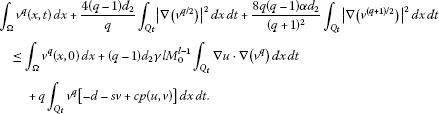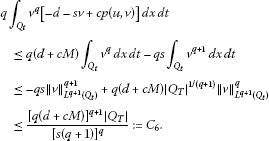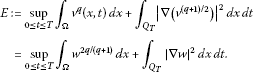Theory and Modern Applications

# Global solutions for a general predator-prey model with cross-diffusion effects

## Abstract

This work investigates a general Lotka-Volterra type predator-prey model with nonlinear cross-diffusion effects representing the tendency of a predator to get closer to prey. Using the energy estimate method, Sobolev embedding theorems and the bootstrap arguments, the existence of global solutions is proved when the space dimension is less than 10.

MSC:35K57, 35B35.

## 1 Introduction

Consider the following predator-prey model with cross-diffusion effects:

$\left\{\begin{array}{cc}{u}_{t}-{d}_{1}\mathrm{\Delta }\left[\left(1+\delta u\right)u\right]=ug\left(u\right)-p\left(u,v\right)v,\hfill & x\in \mathrm{\Omega },t>0,\hfill \\ {v}_{t}-{d}_{2}\mathrm{\Delta }\left[\left(1+\alpha v+\frac{\gamma }{1+{u}^{l}}\right)v\right]=v\left[-d-sv+cp\left(u,v\right)\right],\hfill & x\in \mathrm{\Omega },t>0,\hfill \\ \frac{\partial u}{\partial \nu }=\frac{\partial v}{\partial \nu }=0,\hfill & x\in \partial \mathrm{\Omega },t>0,\hfill \\ u\left(x,0\right)={u}_{0}\left(x\right)\ge \left(\not\equiv \right)0,\phantom{\rule{2em}{0ex}}v\left(x,0\right)={v}_{0}\left(x\right)\ge \left(\not\equiv \right)0,\hfill & x\in \mathrm{\Omega },\hfill \end{array}$
(1)

where $\mathrm{\Omega }\subset {\mathbb{R}}^{n}$ ($n\ge 1$) is a bounded domain with smooth boundary Ω, ν is the outward unit normal vector of the boundary Ω, the given coefficients d, s, c, ${d}_{1}$, ${d}_{2}$, δ, α, γ and l are positive constants. The functions $g\in {C}^{1}\left(\left[0,\mathrm{\infty }\right)\right)$ and $p\in C\left(\left[0,\mathrm{\infty }\right)×\left[0,\mathrm{\infty }\right)\right)$ are assumed to satisfy the following two hypotheses throughout this paper:

(H1) $g\left(0\right)>0$ and ${g}^{\prime }\left(u\right)<0$ for all $u>0$, and there exists a positive constant K such that $g\left(K\right)=0$.

(H2) For all $u,v\ge 0$, $0\le p\left(u,v\right)\le Ch\left(u\right)$ for some positive constant C and a continuous function $h\left(u\right)$.

In the model (1), u and v represent the densities of the prey and predator respectively, the constants d, s and c refer to the predator death rate, the inter-specific competition coefficient and the conversion rate of the prey to the predator, and $g\left(u\right)$ represents the growth rate of the prey in the absence of the predator. The function $p\left(u,v\right)$ is called the functional response of the predator to the prey. The constants ${d}_{1}$ and ${d}_{2}$ are the diffusion rates of the prey and predator respectively, δ and α are referred to as self-diffusion pressures, and the term ${d}_{2}\mathrm{\Delta }\left[\gamma v/\left(1+{u}^{l}\right)\right]$ in the predator equation is cross-diffusion pressure, which expresses the population flux of the predator resulting from the presence of prey species. Biologically, this term represents the tendency of the predator to chase its prey. It is clear that such an environment of prey-predator interaction often occurs in reality. For example, in , with the similar biological interpretation, the authors also introduced the same cross-diffusion term as in (1) to the predator of various predator-prey models.

Mathematically, one of the most important problem for (1) is to establish the existence of global solutions. However, to our knowledge, there are only a few works. In , the global existence result was shown without any restrictions on space dimensions by H. Li, P. Pang and M. Wang. But their results are not valid for (1) because some restrictions are required for the term $p\left(u,v\right)v/u$, so that the standard reaction term like Lotka-Volterra type is excluded in their works. The purpose of this paper is to establish a sufficient condition for the existence of global solutions for (1) without any restrictions on the term $p\left(u,v\right)v/u$ in the higher dimensional case. Our main result is as follows.

Theorem 1.1 Let $l\ge 1$ and $n<10$. Assume that ${u}_{0}$, ${v}_{0}$ satisfy the zero Neumann boundary condition and belong to ${C}^{2+\lambda }\left(\overline{\mathrm{\Omega }}\right)$ for some $0<\lambda <1$. Then (1) possesses a unique non-negative solution $\left(u,v\right)$, such that $u\in {C}^{2+\lambda ,\left(2+\lambda \right)/2}\left(\overline{\mathrm{\Omega }}×\left[0,\mathrm{\infty }\right)\right)$ and $v\in {C}^{1+\lambda ,\left(1+\lambda \right)/2}\left(\overline{\mathrm{\Omega }}×\left[0,\mathrm{\infty }\right)\right)$. Moreover, if $l=1$ or $l\ge 2$, then $v\in {C}^{2+\lambda ,\left(2+\lambda \right)/2}\left(\overline{\mathrm{\Omega }}×\left[0,\mathrm{\infty }\right)\right)$.

As to the stationary problem for (1), there have been some works, for example, see [1, 2, 47] and references therein.

The paper is organized as follows. In Section 2, we present some known results and prove some preliminary results that are used in Section 3. In Section 3, we establish ${L}^{p}$-estimates for v. In Section 4, we establish ${L}^{\mathrm{\infty }}$-estimates for v and give a proof of Theorem 1.1.

## 2 Preliminaries

For the time-dependent solutions of (1), the local existence is an immediate consequence of . The results can be summarized as follows.

Theorem A Let ${u}_{0}$, ${v}_{0}\in {W}_{p}^{1}\left(\mathrm{\Omega }\right)$, where $p>n$, be nonnegative functions. Then the system (1) has a unique nonnegative solution $\left(u,v\right)$ such that $u,v\in C\left(\left[0,T\right),{W}_{p}^{1}\left(\mathrm{\Omega }\right)\right)\cap {C}^{\mathrm{\infty }}\left(\left(0,T\right),{C}^{\mathrm{\infty }}\left(\mathrm{\Omega }\right)\right)$, where $T\in \left(0,+\mathrm{\infty }\right]$ is the maximal existence time of the solution. If the solution $\left(u,v\right)$ satisfies the estimate

$sup\left\{{\parallel u\left(\cdot ,t\right)\parallel }_{{W}_{p}^{1}\left(\mathrm{\Omega }\right)},{\parallel v\left(\cdot ,t\right)\parallel }_{{W}_{p}^{1}\left(\mathrm{\Omega }\right)}:t\in \left(0,T\right)\right\}<\mathrm{\infty },$

then $T=+\mathrm{\infty }$. If, in addition, ${u}_{0},{v}_{0}\in {W}_{p}^{2}\left(\mathrm{\Omega }\right)$, then $u,v\in C\left(\left[0,\mathrm{\infty }\right),{W}_{p}^{2}\left(\mathrm{\Omega }\right)\right)$.

Let

$\begin{array}{c}{Q}_{T}=\mathrm{\Omega }×\left[0,T\right),\hfill \\ {\parallel u\parallel }_{{L}^{p,q}\left({Q}_{T}\right)}={\left({\int }_{0}^{T}{\left({\int }_{\mathrm{\Omega }}{|u\left(x,t\right)|}^{p}\phantom{\rule{0.2em}{0ex}}dx\right)}^{\frac{q}{p}}\phantom{\rule{0.2em}{0ex}}dt\right)}^{1/q},\phantom{\rule{1em}{0ex}}{L}^{p}\left({Q}_{T}\right)={L}^{p,p}\left({Q}_{T}\right),\hfill \\ {\parallel u\parallel }_{{W}_{p}^{2,1}\left({Q}_{T}\right)}={\parallel u\parallel }_{{L}^{p}\left({Q}_{T}\right)}+{\parallel {u}_{t}\parallel }_{{L}^{p}\left({Q}_{T}\right)}+{\parallel \mathrm{\nabla }u\parallel }_{{L}^{p}\left({Q}_{T}\right)}+{\parallel {\mathrm{\nabla }}^{2}u\parallel }_{{L}^{p}\left({Q}_{T}\right)},\hfill \\ {\parallel u\parallel }_{{V}_{2}\left({Q}_{T}\right)}=\underset{0\le t\le T}{sup}{\parallel u\left(\cdot ,t\right)\parallel }_{{L}^{2}\left(\mathrm{\Omega }\right)}+{\parallel \mathrm{\nabla }u\left(x,t\right)\parallel }_{{L}^{2}\left({Q}_{T}\right)}.\hfill \end{array}$

Lemma 2.1 (i) Let $\left(u,v\right)$ be a solution of (1) in $\left[0,T\right)$. There exists a positive constant ${M}_{0}=max\left\{K,{\parallel {u}_{0}\parallel }_{{L}^{\mathrm{\infty }}\left(\mathrm{\Omega }\right)}\right\}$ such that $0\le u\le {M}_{0}$ and $v\ge 0$.

(ii) For any $T>0$, there exist two positive constants ${C}_{1}\left(T\right)$ and ${C}_{2}\left(T\right)$ such that

$\underset{0\le t\le T}{sup}{\parallel v\left(\cdot ,t\right)\parallel }_{{L}^{1}\left(\mathrm{\Omega }\right)}\le {C}_{1}\left(T\right),\phantom{\rule{1em}{0ex}}{\parallel v\parallel }_{{L}^{2}\left({Q}_{T}\right)}\le {C}_{2}\left(T\right).$

(iii) For any $T>0$, there exists a positive constant ${C}_{3}\left(T\right)$ such that ${\parallel \mathrm{\nabla }u\parallel }_{{L}^{4}\left({Q}_{T}\right)}\le {C}_{3}\left(T\right)$.

Proof (i) Applying the maximum principle to (1), we have the result (i).

1. (ii)

Integrating the second equation of (1) over the domain Ω and by (i) and (H2), we have

$\begin{array}{rcl}\frac{d}{dt}{\int }_{\mathrm{\Omega }}v\phantom{\rule{0.2em}{0ex}}dx& =& {\int }_{\mathrm{\Omega }}v\left[-d-sv+cp\left(u,v\right)\right]\phantom{\rule{0.2em}{0ex}}dx\\ \le & \left(d+cM\right){\int }_{\mathrm{\Omega }}v\phantom{\rule{0.2em}{0ex}}dx-s{\int }_{\mathrm{\Omega }}{v}^{2}\phantom{\rule{0.2em}{0ex}}dx\\ \le & \left(d+cM\right){\int }_{\mathrm{\Omega }}v\phantom{\rule{0.2em}{0ex}}dx-\frac{s}{|\mathrm{\Omega }|}{\left({\int }_{\mathrm{\Omega }}v\phantom{\rule{0.2em}{0ex}}dx\right)}^{2}.\end{array}$

Then we have ${sup}_{0\le t\le T}{\parallel v\left(\cdot ,t\right)\parallel }_{{L}^{1}\left(\mathrm{\Omega }\right)}\le {C}_{1}\left(T\right)$. Integrating the above inequality from 0 to T, we have

$s{\parallel v\parallel }_{{L}^{2}\left({Q}_{T}\right)}^{2}\le \left(d+cM\right)T{C}_{1}\left(T\right)+{\parallel {v}_{0}\parallel }_{{L}^{1}\left(\mathrm{\Omega }\right)}.$

Therefore, ${\parallel v\parallel }_{{L}^{2}\left({Q}_{T}\right)}\le {C}_{2}\left(T\right)$.

1. (iii)

Let ${w}_{1}=\left(1+\delta u\right)u$, then ${w}_{1}$ satisfies the equation

${w}_{1t}={d}_{1}\left(1+2\delta u\right)\mathrm{\Delta }{w}_{1}+{c}_{1}+{c}_{2}v,$

where ${c}_{1}=\left(1+2\delta u\right)ug\left(u\right)$ and ${c}_{2}=-\left(1+2\delta u\right)p\left(u,v\right)$. Since u, ${c}_{1}$, ${c}_{2}$ are bounded and $v\in {L}^{2}\left({Q}_{T}\right)$, we can prove ${w}_{1}\in {W}_{2}^{2,1}\left({Q}_{T}\right)$ in the same way as Lemma 2.4 in . Moreover, ${w}_{1}$ satisfies

${w}_{1t}\le {d}_{1}\left(1+2\delta u\right)\mathrm{\Delta }{w}_{1}+\left(1+2\delta u\right)ug\left(u\right)={d}_{1}\sqrt{1+4\delta {w}_{1}}\mathrm{\Delta }{w}_{1}+\left(1+2\delta u\right)ug\left(u\right).$

Applying Proposition 2.1 in , we obtain $\mathrm{\nabla }{w}_{1}\in {L}^{4}\left({Q}_{T}\right)$, which in turn implies ${\parallel \mathrm{\nabla }u\parallel }_{{L}^{4}\left({Q}_{T}\right)}\le {C}_{3}\left(T\right)$. □

In order to establish ${L}^{p}$-estimates for solutions of (1), we need the following result which can be found in .

Lemma 2.2 Let $q>1$ and $\stackrel{˜}{q}=2+4q/\left[n\left(q+1\right)\right]$. Suppose that w satisfies

$\underset{0\le t\le T}{sup}{\parallel w\parallel }_{{L}^{2q/\left(q+1\right)}\left(\mathrm{\Omega }\right)}+{\parallel \mathrm{\nabla }w\parallel }_{{L}^{2}\left({Q}_{T}\right)}<\mathrm{\infty },$

and there exist positive constants $\stackrel{˜}{\beta }\in \left(0,1\right)$ and ${C}_{T}>0$ such that ${\int }_{\mathrm{\Omega }}{|w\left(\cdot ,t\right)|}^{\stackrel{˜}{\beta }}\phantom{\rule{0.2em}{0ex}}dx\le {C}_{T}$ for all $t\in \left[0,T\right]$. Then there exists a positive constant ${M}^{\prime }$ independent of w but depending on n, Ω, q, $\stackrel{˜}{\beta }$ and ${C}_{T}$, such that

${\parallel w\parallel }_{{L}^{\stackrel{˜}{q}}\left({Q}_{T}\right)}\le {M}^{\prime }\left\{1+{\left(\underset{0\le t\le T}{sup}{\parallel w\left(t\right)\parallel }_{{L}^{2q/\left(q+1\right)}\left(\mathrm{\Omega }\right)}\right)}^{4q/\left[n\left(q+1\right)\stackrel{˜}{q}\right]}{\parallel \mathrm{\nabla }w\parallel }_{{L}^{2}\left({Q}_{T}\right)}^{2/\stackrel{˜}{q}}\right\}.$

## 3 ${L}^{p}$-estimates for v

Let $t\in \left(0,T\right]$, ${Q}_{t}=\mathrm{\Omega }×\left(0,t\right)$. In this section, ${C}_{1},{C}_{2},\dots$ and ${M}_{1},{M}_{2},\dots$ shall denote positive constants that may depend on Ω, q, n, the coefficients in (1), T (but not on t) and the following norms ${\parallel {u}_{0}\parallel }_{{W}_{q}^{2-2/q}}$, ${\parallel {u}_{0}\parallel }_{{L}^{\mathrm{\infty }}\left(\mathrm{\Omega }\right)}$ and ${\parallel {v}_{0}\parallel }_{{L}^{q}\left(\mathrm{\Omega }\right)}$.

Lemma 3.1 There exist positive constants ${C}_{4}$ and ${C}_{5}$ such that ${\parallel v\parallel }_{{V}_{2}\left({Q}_{T}\right)}\le {C}_{4}$ and ${\parallel v\parallel }_{{L}^{p}\left({Q}_{T}\right)}\le {C}_{5}$ if $p<4\left(n+1\right)/{\left(n-2\right)}_{+}$, where ${a}_{+}=max\left\{a,0\right\}$.

Proof For any constant $q>1$, multiplying the second equation of (1) by $q{v}^{q-1}$ and using the integration by parts, we obtain

$\begin{array}{rcl}\frac{d}{dt}{\int }_{\mathrm{\Omega }}{v}^{q}dx& =& q{d}_{2}{\int }_{\mathrm{\Omega }}{v}^{q-1}\mathrm{\nabla }\cdot \left[\left(1+2\alpha v+\frac{\gamma }{1+{u}^{l}}\right)\mathrm{\nabla }v-\frac{\gamma l{u}^{l-1}v}{{\left(1+{u}^{l}\right)}^{2}}\mathrm{\nabla }u\right]\phantom{\rule{0.2em}{0ex}}dx\\ +q{\int }_{\mathrm{\Omega }}{v}^{q}\left[-d-sv+cp\left(u,v\right)\right]\phantom{\rule{0.2em}{0ex}}dx\\ =& -q\left(q-1\right){d}_{2}{\int }_{\mathrm{\Omega }}{v}^{q-2}\left(1+2\alpha v+\frac{\gamma }{1+{u}^{l}}\right){|\mathrm{\nabla }v|}^{2}\phantom{\rule{0.2em}{0ex}}dx\\ +\left(q-1\right){d}_{2}\gamma l{\int }_{\mathrm{\Omega }}\frac{{u}^{l-1}}{{\left(1+{u}^{l}\right)}^{2}}\mathrm{\nabla }u\cdot \mathrm{\nabla }\left({v}^{q}\right)\phantom{\rule{0.2em}{0ex}}dx+q{\int }_{\mathrm{\Omega }}{v}^{q}\left[-d-sv+cp\left(u,v\right)\right]\phantom{\rule{0.2em}{0ex}}dx\\ \le & -q\left(q-1\right){d}_{2}{\int }_{\mathrm{\Omega }}{v}^{q-2}{|\mathrm{\nabla }v|}^{2}\phantom{\rule{0.2em}{0ex}}dx-2\alpha q\left(q-1\right){d}_{2}{\int }_{\mathrm{\Omega }}{v}^{q-1}{|\mathrm{\nabla }v|}^{2}\phantom{\rule{0.2em}{0ex}}dx\\ +\left(q-1\right){d}_{2}\gamma l{\int }_{\mathrm{\Omega }}\frac{{u}^{l-1}}{{\left(1+{u}^{l}\right)}^{2}}\mathrm{\nabla }u\cdot \mathrm{\nabla }\left({v}^{q}\right)\phantom{\rule{0.2em}{0ex}}dx+q{\int }_{\mathrm{\Omega }}{v}^{q}\left[-d-sv+cp\left(u,v\right)\right]\phantom{\rule{0.2em}{0ex}}dx\\ =& -\frac{4\left(q-1\right){d}_{2}}{q}{\int }_{\mathrm{\Omega }}{|\mathrm{\nabla }\left({v}^{q/2}\right)|}^{2}\phantom{\rule{0.2em}{0ex}}dx-\frac{8q\left(q-1\right)\alpha {d}_{2}}{{\left(q+1\right)}^{2}}{\int }_{\mathrm{\Omega }}{|\mathrm{\nabla }\left({v}^{\left(q+1\right)/2}\right)|}^{2}\phantom{\rule{0.2em}{0ex}}dx\\ +\left(q-1\right){d}_{2}\gamma l{M}_{0}^{l-1}{\int }_{\mathrm{\Omega }}\mathrm{\nabla }u\cdot \mathrm{\nabla }\left({v}^{q}\right)\phantom{\rule{0.2em}{0ex}}dx+q{\int }_{\mathrm{\Omega }}{v}^{q}\left[-d-sv+cp\left(u,v\right)\right]\phantom{\rule{0.2em}{0ex}}dx.\end{array}$
(2)

Integrating (2) from 0 to t, we have(3)

The last term in (3) may be estimated by(4)

Let ${r}_{0}=4$ and ${p}_{0}=2{r}_{0}/\left({r}_{0}-2\right)$. Since $1/{r}_{0}+1/2+1/{p}_{0}=1$ and u is in ${L}^{{r}_{0}}\left({Q}_{T}\right)$, using the Hölder’s inequality, we have

$\begin{array}{rcl}|{\int }_{{Q}_{t}}\mathrm{\nabla }u\cdot \mathrm{\nabla }\left({v}^{q}\right)\phantom{\rule{0.2em}{0ex}}dx\phantom{\rule{0.2em}{0ex}}dt|& =& \frac{2q}{q+1}|{\int }_{{Q}_{t}}{v}^{\left(q-1\right)/2}\cdot \mathrm{\nabla }\left({v}^{\left(q+1\right)/2}\right)\cdot \mathrm{\nabla }u\phantom{\rule{0.2em}{0ex}}dx\phantom{\rule{0.2em}{0ex}}dt|\\ \le & \frac{2q}{q+1}{\parallel v\parallel }_{{L}^{{p}_{0}\left(q-1\right)/2}\left({Q}_{t}\right)}^{\left(q-1\right)/2}\cdot {\parallel \mathrm{\nabla }\left({v}^{\left(q+1\right)/2}\right)\parallel }_{{L}^{2}\left({Q}_{t}\right)}\cdot {\parallel \mathrm{\nabla }u\parallel }_{{L}^{{r}_{0}}\left({Q}_{t}\right)}\\ \le & \frac{2q{C}_{3}}{q+1}{\parallel v\parallel }_{{L}^{{p}_{0}\left(q-1\right)/2}\left({Q}_{t}\right)}^{\left(q-1\right)/2}\cdot {\parallel \mathrm{\nabla }\left({v}^{\left(q+1\right)/2}\right)\parallel }_{{L}^{2}\left({Q}_{t}\right)}.\end{array}$
(5)

The substitution (4) and (5) into (3) leads to

$\begin{array}{c}{\int }_{\mathrm{\Omega }}{v}^{q}\left(x,t\right)\phantom{\rule{0.2em}{0ex}}dx+\frac{4\left(q-1\right){d}_{2}}{q}{\int }_{{Q}_{t}}{|\mathrm{\nabla }\left({v}^{q/2}\right)|}^{2}\phantom{\rule{0.2em}{0ex}}dx\phantom{\rule{0.2em}{0ex}}dt+\frac{8q\left(q-1\right)\alpha {d}_{2}}{{\left(q+1\right)}^{2}}{\int }_{{Q}_{t}}{|\mathrm{\nabla }\left({v}^{\left(q+1\right)/2}\right)|}^{2}\phantom{\rule{0.2em}{0ex}}dx\phantom{\rule{0.2em}{0ex}}dt\hfill \\ \phantom{\rule{1em}{0ex}}\le {C}_{7}+{C}_{8}{\parallel v\parallel }_{{L}^{{p}_{0}\left(q-1\right)/2}\left({Q}_{t}\right)}^{\left(q-1\right)/2}\cdot {\parallel \mathrm{\nabla }\left({v}^{\left(q+1\right)/2}\right)\parallel }_{{L}^{2}\left({Q}_{t}\right)}\hfill \\ \phantom{\rule{1em}{0ex}}\le {C}_{7}+\frac{{C}_{8}}{4\epsilon }{\parallel v\parallel }_{{L}^{{p}_{0}\left(q-1\right)/2}\left({Q}_{t}\right)}^{q-1}+{C}_{8}\epsilon {\parallel \mathrm{\nabla }\left({v}^{\left(q+1\right)/2}\right)\parallel }_{{L}^{2}\left({Q}_{t}\right)}^{2}\hfill \end{array}$

for any $\epsilon >0$. By choosing a sufficiently small ε, such that ${C}_{8}\epsilon <8q\left(q-1\right)\alpha {d}_{2}/{\left(q+1\right)}^{2}$, we have

${\parallel v\left(\cdot ,t\right)\parallel }_{{L}^{q}\left(\mathrm{\Omega }\right)}^{q}+{\parallel \mathrm{\nabla }\left({v}^{q/2}\right)\parallel }_{{L}^{2}\left({Q}_{t}\right)}^{2}+{\parallel \mathrm{\nabla }\left({v}^{\left(q+1\right)/2}\right)\parallel }_{{L}^{2}\left({Q}_{t}\right)}^{2}\le {C}_{9}\left(1+{\parallel v\parallel }_{{L}^{{p}_{0}\left(q-1\right)/2}\left({Q}_{t}\right)}^{q-1}\right),$
(6)

where ${C}_{9}=max\left\{{C}_{7},{C}_{8}/\left(4\epsilon \right)\right\}$. Setting $w={v}^{\left(q+1\right)/2}$, we defineSo, from (6), we have

$E+{\parallel \mathrm{\nabla }\left({v}^{q/2}\right)\parallel }_{{L}^{2}\left({Q}_{T}\right)}^{2}\le {C}_{9}\left(1+{\parallel w\parallel }_{{L}^{{p}_{0}\left(q-1\right)/\left(q+1\right)}\left({Q}_{T}\right)}^{2\left(q-1\right)/\left(q+1\right)}\right).$
(7)

For any $q>1$, if

$\left(n{p}_{0}-2n-4\right)q\le \left(2+{p}_{0}\right)n,$
(8)

then, ${p}_{0}\left(q-1\right)/\left(q+1\right)\le \stackrel{˜}{q}=2+4q/\left[n\left(q+1\right)\right]$. Therefore, by the Hölder’s inequality, we have

${\parallel w\parallel }_{{L}^{{p}_{0}\left(q-1\right)/\left(q+1\right)}\left({Q}_{T}\right)}\le {C}_{10}{\parallel w\parallel }_{{L}^{\stackrel{˜}{q}}\left({Q}_{T}\right)},$
(9)

where ${C}_{10}={|{Q}_{T}|}^{\left(q+1\right)/\left[{p}_{0}\left(q-1\right)\right]-1/\stackrel{˜}{q}}$. Setting $\stackrel{˜}{\beta }=2/\left(q+1\right)\in \left(0,1\right)$, by Lemma 2.1(ii), we have

${\parallel w\left(\cdot ,t\right)\parallel }_{{L}^{\stackrel{˜}{\beta }}\left(\mathrm{\Omega }\right)}={\parallel v\left(\cdot ,t\right)\parallel }_{{L}^{1}\left(\mathrm{\Omega }\right)}^{1/\stackrel{˜}{\beta }}\le {\left[{C}_{1}\left(T\right)\right]}^{1/\stackrel{˜}{\beta }},\phantom{\rule{1em}{0ex}}\mathrm{\forall }t\in \left[0,T\right).$
(10)

Therefore, by (10), Lemma 2.2 and the definition of E, the expression (9) yields

${\parallel w\parallel }_{{L}^{{p}_{0}\left(q-1\right)/\left(q+1\right)}\left({Q}_{T}\right)}\le {C}_{10}{\parallel w\parallel }_{{L}^{\stackrel{˜}{q}}\left({Q}_{T}\right)}\le {C}_{10}{M}^{\prime }\left\{1+{E}^{2/\left(n\stackrel{˜}{q}\right)}{E}^{1/\stackrel{˜}{q}}\right\}.$
(11)

Then, by (7) and (11), we have

$E\le {C}_{11}\left(1+{E}^{\mu }{E}^{\nu }\right)$
(12)

with

$\mu =\frac{4\left(q-1\right)}{n\stackrel{˜}{q}\left(q+1\right)},\phantom{\rule{2em}{0ex}}\nu =\frac{2\left(q-1\right)}{\stackrel{˜}{q}\left(q+1\right)}.$

Since

$\mu +\nu =\frac{2\left(q-1\right)}{\stackrel{˜}{q}\left(q+1\right)}\left(\frac{2}{n}+1\right)<\frac{1}{\stackrel{˜}{q}}\left[\frac{4q}{n\left(q+1\right)}+2\right]=1,$

it follows from (12) that there exists a positive constant ${C}_{12}$ such that $E\le {C}_{12}$. By (11) and (12) we get $w\in {L}^{\stackrel{˜}{q}}\left({Q}_{T}\right)$ which in turn implies that $v\in {L}^{p}\left({Q}_{T}\right)$ with $p=\stackrel{˜}{q}\left(q+1\right)/2$ for any q satisfying (8). Now, looking at (8), if $n\le 2$, we have

$n{p}_{0}-2n-4=2\left(n-2\right)\le 0,$

then (8) holds for all q. So for $n\le 2$, $v\in {L}^{p}\left({Q}_{T}\right)$ for all $p>1$. If $n>2$, then (8) is equivalent to

$1

Then

$\frac{\stackrel{˜}{q}\left(q+1\right)}{2}=q+1+\frac{2q}{n}\le {\overline{r}}_{1}:={q}_{0}+1+\frac{2{q}_{0}}{n}=\frac{4\left(n+1\right)}{n-2}.$

So, we see that v is in ${L}^{p}\left({Q}_{T}\right)$ for all $1. Since (8) holds true for $q=2$. So E is bounded for $q=2$. It follows from (7) and (11) that ${\parallel v\parallel }_{{V}_{2}\left({Q}_{T}\right)}$ is bounded for any n. Namely, there exist positive constants ${C}_{4}$ and ${C}_{5}$ such that ${\parallel v\parallel }_{{V}_{2}\left({Q}_{T}\right)}\le {C}_{4}$ for any n, and ${\parallel v\parallel }_{{L}^{p}\left({Q}_{T}\right)}\le {C}_{5}$ for $p<4\left(n+1\right)/{\left(n-2\right)}_{+}$. □

## 4 ${L}^{\mathrm{\infty }}$-estimates for v and a proof of Theorem 1.1

Lemma 4.1 Suppose that there are positive constants ${r}_{1}>max\left\{\left(n+2\right)/2,3\right\}$ and ${C}_{{r}_{1}}$ such that ${\parallel v\parallel }_{{L}^{{r}_{1}}\left({Q}_{T}\right)}\le {C}_{{r}_{1}}$. Then, $v\in {L}^{p}\left({Q}_{T}\right)$ for any $p>1$.

Proof First, the equation of u can be written in the divergence form as

${u}_{t}={d}_{1}\mathrm{\nabla }\cdot \left[\left(1+2\delta u\right)\mathrm{\nabla }u\right]+ug\left(u\right)-p\left(u,v\right)v,$

where $1+2\delta u$ is bounded in ${\overline{Q}}_{T}$ and $ug\left(u\right)-p\left(u,v\right)v$ is in ${L}^{{r}_{1}}$ with ${r}_{1}>\left(n+2\right)/2$. Application of the Hölder continuity result (see , Theorem 10.1, p.204) yields

By similar arguments as in the proof of Lemma 2.1(iii), we see that ${w}_{1}=\left(1+\delta u\right)u\in {W}_{{r}_{1}}^{2,1}\left({Q}_{T}\right)$. This implies $\mathrm{\nabla }u=\mathrm{\nabla }{w}_{1}/\left(1+2\delta u\right)$ in ${L}^{{r}_{1}}\left({Q}_{T}\right)$. Replacing ${r}_{0}$ by ${r}_{1}$ and ${p}_{0}$ by ${p}_{1}$ in the proof of Lemma 3.1, we see that either $v\in {L}^{p}\left({Q}_{T}\right)$ for any $p>1$ or else $v\in {L}^{{r}_{2}}\left({Q}_{T}\right)$ with ${r}_{2}:=\left(n+1\right){r}_{1}/\left(n+2-{r}_{1}\right)$. The latter case happens if and only if

$n+2-{r}_{1}>0.$

If $v\in {L}^{{r}_{2}}\left({Q}_{T}\right)$, applying Theorem 9.1 in  and its remark (p.351) again, we have u in ${L}^{{r}_{2}}\left({Q}_{T}\right)$. Repeating the above steps, we will get the sequence of numbers

${r}_{k+1}:=\frac{\left(n+1\right){r}_{k}}{n+2-{r}_{k}}.$

We stop and get the conclusion that $v\in {L}^{p}\left({Q}_{T}\right)$ for any $p>1$ when

$n+2-{r}_{k}\le 0.$
(13)

It is not hard to verify by inducting that ${r}_{k}>3$, $k=1,2,\dots$ , and

$\frac{{r}_{k+1}}{{r}_{k}}=\frac{n+1}{n+2-{r}_{k}}\ge \frac{n+1}{n-1}>1.$

Therefore, (13) must hold for some k. We stop at this k and conclude that $v\in {L}^{p}\left({Q}_{T}\right)$ for any $p>1$. □

Notice that $\left(n+2\right)/2<4\left(n+1\right)/{\left(n-2\right)}_{+}$ for $n<10$. Therefore, by Lemmas 3.1 and 4.1, we know that $v\in {V}_{2}\left({Q}_{T}\right)$ and ${L}^{p}\left({Q}_{T}\right)$ for any $p>1$. More precisely, there exists a positive constant $\overline{M}$ depending only on T, Ω, the coefficients of (1) and initial conditions ${u}_{0}$, ${v}_{0}$, but not on t, such that

On the basis of the above results, we may demonstrate a proof of Theorem 1.1 analogous to that in the paper .

Proof of Theorem 1.1 Since $v\in {L}^{q}\left({Q}_{T}\right)$ for any $q>1$ if $n<10$, we have ${w}_{1}=\left(1+\delta u\right)u\in {W}_{q}^{2,1}\left({Q}_{T}\right)$. Hence it follows from the Sobolev embedding theorem that ${w}_{1}\in {C}^{1+{\beta }^{\ast },\left(1+{\beta }^{\ast }\right)/2}\left({\overline{Q}}_{T}\right)$ for any ${\beta }^{\ast }\in \left(0,1\right)$, which in turn implies

$u\in {C}^{1+{\beta }^{\ast },\left(1+{\beta }^{\ast }\right)/2}\left({\overline{Q}}_{T}\right).$
(14)

The second equation in (1) can be rewritten as

${v}_{t}=\mathrm{\nabla }\cdot \left[{d}_{2}\left(1+2\alpha v+\frac{\gamma }{1+{u}^{l}}\right)\mathrm{\nabla }v-\frac{{d}_{2}\gamma l{u}^{l-1}v}{{\left(1+{u}^{l}\right)}^{2}}\mathrm{\nabla }u\right]+v\left[-d-sv+cp\left(u,v\right)\right].$

Since $v\left[-d-sv+cp\left(u,v\right)\right]\in {L}^{\mathrm{\infty }}\left({Q}_{T}\right)$ by the assumption H2, Lemmas 2.1 and 4.1, and v, $\gamma /\left(1+{u}^{l}\right)$ and ${d}_{2}\gamma l{u}^{l-1}\mathrm{\nabla }u/{\left(1+{u}^{l}\right)}^{2}$ are all bounded for $l\ge 1$, the Hölder estimates  show that

(15)

Rewrite the first equation in (1) as

${u}_{t}={d}_{1}\left(1+2\delta u\right)\mathrm{\Delta }u+2{d}_{1}\delta {|\mathrm{\nabla }u|}^{2}+ug\left(u\right)-p\left(u,v\right)v.$

From (14) and (15), it follows that ${d}_{1}\left(1+2\delta u\right)$ and $2{d}_{1}\delta {|\mathrm{\nabla }u|}^{2}+ug\left(u\right)-p\left(u,v\right)v$ are in ${C}^{\stackrel{˜}{\sigma },\stackrel{˜}{\sigma }/2}\left({\overline{Q}}_{T}\right)$, where $\stackrel{˜}{\sigma }=min\left\{{\beta }^{\ast },\sigma \right\}$. Applying the Schauder estimates, we have

$u\in {C}^{2+{\sigma }^{\ast },\left(2+{\sigma }^{\ast }\right)/2}\left({\overline{Q}}_{T}\right),\phantom{\rule{2em}{0ex}}{\sigma }^{\ast }=min\left\{\stackrel{˜}{\sigma },\lambda \right\}.$
(16)

In order to derive the regularity of v, it is convenient to introduce the function

${w}_{2}=\left(1+\alpha v+\frac{\gamma }{1+{u}^{l}}\right)v,$
(17)

which satisfies

$\frac{\partial {w}_{2}}{\partial t}={d}_{2}\left(1+2\alpha v+\frac{\gamma }{1+{u}^{l}}\right)\mathrm{\Delta }{w}_{2}+{h}^{\ast }\left(x,t\right)$
(18)

with

${h}^{\ast }\left(x,t\right)=v\left(1+2\alpha v+\frac{\gamma }{1+{u}^{l}}\right)\left[d-sv+cp\left(u,v\right)\right]-\frac{\gamma l{u}^{l-1}v}{{\left(1+{u}^{l}\right)}^{2}}{u}_{t}.$

Note that ${d}_{2}\left(1+2\alpha v+\gamma /\left(1+{u}^{l}\right)\right)$ and ${h}^{\ast }\left(x,t\right)$ are in ${C}^{{\sigma }^{\ast },{\sigma }^{\ast }/2}\left({\overline{Q}}_{T}\right)$, thus, an application of the Schauder estimates to (18) gives

${w}_{2}\in {C}^{2+{\sigma }^{\ast },\left(2+{\sigma }^{\ast }\right)/2}\left({\overline{Q}}_{T}\right).$

Then (17) and $l\ge 1$ imply that

$v\in {C}^{1+{\sigma }^{\ast },\left(1+{\sigma }^{\ast }\right)/2}\left({\overline{Q}}_{T}\right).$
(19)

Here, it is not hard to see that for the case $l=1$ or the case $l\ge 2$,

$v\in {C}^{2+{\sigma }^{\ast },\left(2+{\sigma }^{\ast }\right)/2}\left({\overline{Q}}_{T}\right).$
(20)

When $\lambda \le {\sigma }^{\ast }$, ${\sigma }^{\ast }=\lambda$. When ${\sigma }^{\ast }<\lambda$, repeating the above bootstrap arguments by making use of (16) and (19) in place of (14) and (15), one can find that (16) and (19) are fulfilled with ${\sigma }^{\ast }$ replaced by λ.

In the case $l=1$ or $l\ge 2$ the above arguments are also valid if we replace (19) by (20). This completes the proof of Theorem 1.1. □

## References

1. Chen XF, Qi YW, Wang MX: A strongly coupled predator-prey system with non-monotonic functional response. Nonlinear Anal. 2007, 67(6):1966–1979. 10.1016/j.na.2006.08.022

2. Kadota T, Kuto K: Positive steady states for a prey-predator model with some nonlinear diffusion terms. J. Math. Anal. Appl. 2006, 323(2):1387–1401. 10.1016/j.jmaa.2005.11.065

3. Li HL, Pang PYH, Wang MX: Global solutions for a general strongly coupled prey-predator model. Appl. Math. Lett. 2009, 22(10):1508–1512. 10.1016/j.aml.2008.06.050

4. Pang PYH, Wang MX: Strategy and stationary pattern in a three-species predator-prey model. J. Differ. Equ. 2004, 200(2):245–273. 10.1016/j.jde.2004.01.004

5. Wang MX: Stationary patterns of strongly coupled prey-predator models. J. Math. Anal. Appl. 2004, 292(2):484–505. 10.1016/j.jmaa.2003.12.027

6. Wu YP: Existence of stationary solutions with transition layers for a class of cross-diffusion systems. Proc. R. Soc. Edinb. A 2002, 132(6):1493–1511.

7. Zhang LN, Fu SM: Non-constant positive steady states for a predator-prey cross-diffusion model with Beddington-DeAngelis functional response. Bound. Value Probl. 2011., 2011: Article ID 404696

8. Amann H: Dynamic theory of quasilinear parabolic equations-I: abstract evolution equations. Nonlinear Anal. 1988, 12(9):859–919.

9. Amann H: Dynamic theory of quasilinear parabolic equations-II: reaction-diffusion. Differ. Integral Equ. 1990, 3(1):13–75.

10. Amann H: Dynamic theory of quasilinear parabolic equations-III: global existence. Math. Z. 1989, 202(2):219–250. 10.1007/BF01215256

11. Lou Y, Ni WM, Wu YP: On the global existence of a cross-diffusion system. Discrete Contin. Dyn. Syst., Ser. A 1998, 4(2):193–203.

12. Tuoc PV: On global existence of solutions to a cross-diffusion system. J. Math. Anal. Appl. 2008, 343(2):826–834. 10.1016/j.jmaa.2008.01.089

13. Choi YS, Lui R, Yamada Y: Existence of global solutions for the Shigesada-Kawasaki-Teramoto model with strongly coupled cross-diffusion. Discrete Contin. Dyn. Syst. 2004, 10(3):719–730.

14. Ladyzenskaja OA, Solonnikov VA, Ural’ceva NN Translations of Mathematical Monographs 23. In Linear and Quasilinear Partial Differential Equations of Parabolic Type. Am. Math. Soc., Providence; 1968.

## Acknowledgements

This work was supported by the National Natural Science Foundation of China (no. 11061031, 11101334), the Fundamental Research Funds for the Gansu University, and NWNU-LKQN-11-21 Foundations.

## Author information

Authors

### Corresponding author

Correspondence to Lina Zhang.

### Competing interests

The authors declare that they have no competing interests.

### Authors’ contributions

LZ directed the study and carried out the main results of this article. SF established the models and drafted the manuscript. All the authors read and approved the final manuscript.

## Rights and permissions

Reprints and Permissions

Zhang, L., Fu, S. Global solutions for a general predator-prey model with cross-diffusion effects. Adv Differ Equ 2012, 117 (2012). https://doi.org/10.1186/1687-1847-2012-117